# CodeLab - First Steps (Linocut)

## Recommended Posts

You probably know about the technique of linocut to create images.

Let's try to create an effect simulates this technique.

So, step by step.

Step 1. Open any image.Step 2. Apply to him the Outline effect (Effects -> Stylize -> Outline) with default parameters.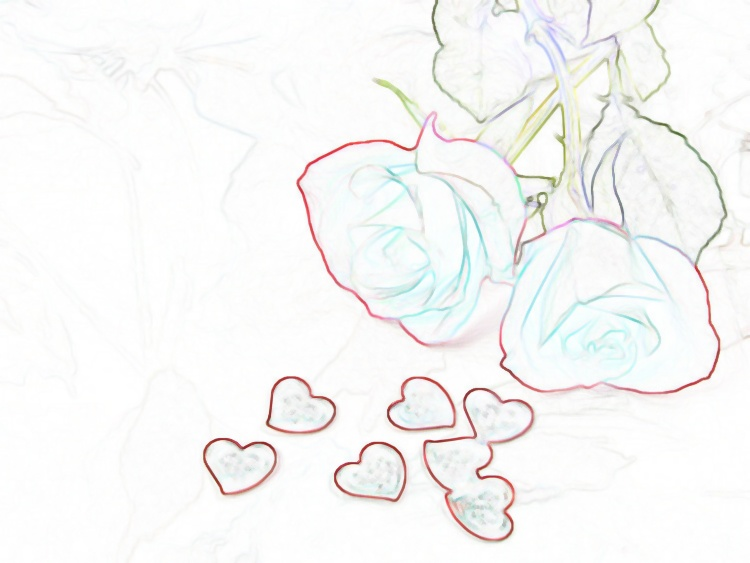Step 3. Set the resulting image in B&W image (Adjustments -> Black and White).

Step 4. In Adjustments menu run the Curves effect (Adjustments-> Curves). Drag the left bottom point of the curve to the right, setting its value to (231.0). Drag the upper-right point of the curve to the left, setting its value to (232.255).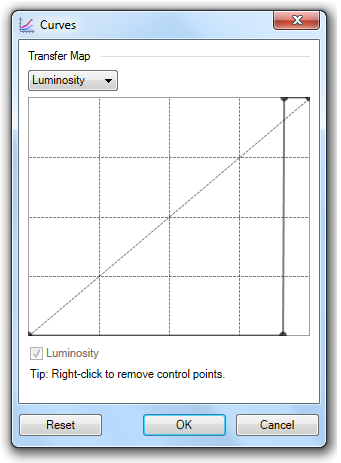Step 5. Invert the image (Adjustments -> Invert Colors). The result is such an image.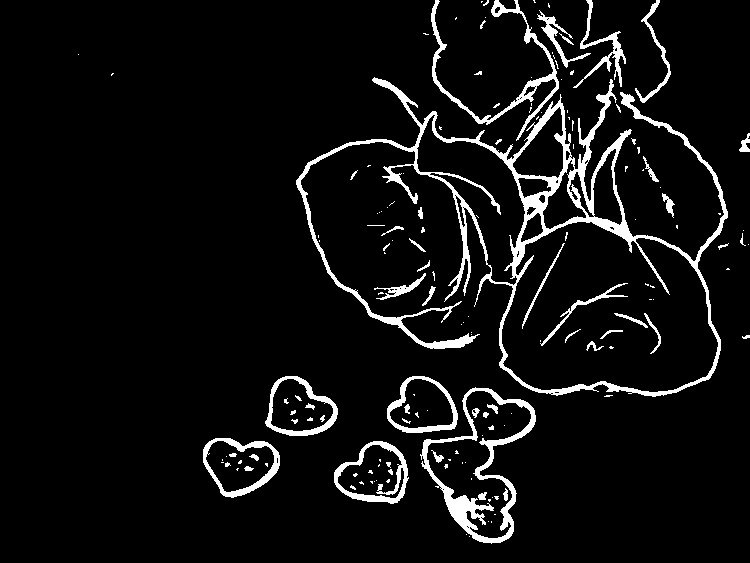Return to Step 4. Set the top point of the curve to (240.255), the lower point - to (215.0). Try to move the left point to the right, to the values (239.0) - you'll notice that this change leads to image detail.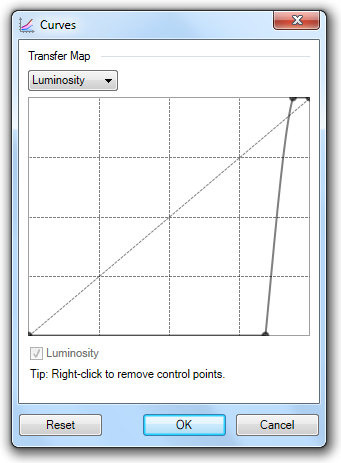Now let's create our effect. Start CodeLab (Effects-> Advanced-> CodeLab). Choose in the menu File -> New. In the drop-down list choose Outline and in the top drop-down list PixelOp choose Desaturate. In the bottom drop-down list PixelOp choose Invert. Click the Generate Code button. We get the following script effect:

```#region UICode
int Amount1=3; // [1,200] Thickness
int Amount2=50; // [0,100] Intensity
#endregion

// Setup for using pixel op
private UnaryPixelOps.Desaturate desaturateOp = new UnaryPixelOps.Desaturate();
private UnaryPixelOps.Invert invertOp = new UnaryPixelOps.Invert();

// Here is the main render loop function
void Render(Surface dst, Surface src, Rectangle rect)
{
// Setup for calling the Outline effect
OutlineEffect outlineEffect = new OutlineEffect();
PropertyCollection outlineProps = outlineEffect.CreatePropertyCollection();
PropertyBasedEffectConfigToken outlineParameters = new PropertyBasedEffectConfigToken(outlineProps);
outlineParameters.SetPropertyValue(OutlineEffect.PropertyNames.Thickness, Amount1);
outlineParameters.SetPropertyValue(OutlineEffect.PropertyNames.Intensity, Amount2);
outlineEffect.SetRenderInfo(outlineParameters, new RenderArgs(dst), new RenderArgs(src));
// Call the Outline function
outlineEffect.Render(new Rectangle { rect }, 0, 1);

// Now in the main render loop, the dst canvas has an outlined version of the src canvas
for (int y = rect.Top; y < rect.Bottom; y++)
{
if (IsCancelRequested) return;
for (int x = rect.Left; x < rect.Right; x++)
{
ColorBgra CurrentPixel = dst[x,y];

CurrentPixel = desaturateOp.Apply(CurrentPixel);

CurrentPixel = invertOp.Apply(CurrentPixel);

dst[x,y] = CurrentPixel;
}
}
}```

In the line

`    outlineParameters.SetPropertyValue(OutlineEffect.PropertyNames.Intensity, Amount2);`

replace Amount2 on 50.

Now let's reproduce a step 4. Between the line

`            CurrentPixel = desaturateOp.Apply(CurrentPixel);`

and line

`            CurrentPixel = invertOp.Apply(CurrentPixel);`

place the following code:

```            int R = CurrentPixel.R;
int G = CurrentPixel.G;
int B = CurrentPixel.B;

// Convert to black and white contrast image
if (R<232) {R=0;}
else R=255;
if (G<232) {G=0;}
else G=255;
if (B<232) {B=0;}
else B=255;

CurrentPixel.R = Int32Util.ClampToByte(R);
CurrentPixel.G = Int32Util.ClampToByte(G);
CurrentPixel.B = Int32Util.ClampToByte(В);```

Are you remember, we changed threshold value of a curve from 215 to 240? Let's reproduce it in our script. Add to the script the integer variable int T = Amount2 + 214. Replace in the code block given above value 232 with a variable T. In the block of codes of the interface rename the Amount1 control to the Tool Size. Rename the Amount2 control to the Details, set its value by default to 17, the minimum value to 1, maximum - to 25.

Now our script will look so:

```#region UICode
int Amount1=3; // [1,200] Tool Size
int Amount2=17; // [1,25] Details
#endregion

// Setup for using pixel op
private UnaryPixelOps.Desaturate desaturateOp = new UnaryPixelOps.Desaturate();
private UnaryPixelOps.Invert invertOp = new UnaryPixelOps.Invert();

// Here is the main render loop function
void Render(Surface dst, Surface src, Rectangle rect)
{
// Setup for calling the Outline effect
OutlineEffect outlineEffect = new OutlineEffect();
PropertyCollection outlineProps = outlineEffect.CreatePropertyCollection();
PropertyBasedEffectConfigToken outlineParameters = new PropertyBasedEffectConfigToken(outlineProps);
outlineParameters.SetPropertyValue(OutlineEffect.PropertyNames.Thickness, Amount1);
outlineParameters.SetPropertyValue(OutlineEffect.PropertyNames.Intensity, Amount2);
outlineEffect.SetRenderInfo(outlineParameters, new RenderArgs(dst), new RenderArgs(src));
// Call the Outline function
outlineEffect.Render(new Rectangle { rect }, 0, 1);

// Now in the main render loop, the dst canvas has an outlined version of the src canvas
for (int y = rect.Top; y < rect.Bottom; y++)
{
if (IsCancelRequested) return;
for (int x = rect.Left; x < rect.Right; x++)
{
ColorBgra CurrentPixel = dst[x,y];

CurrentPixel = desaturateOp.Apply(CurrentPixel);

int R = CurrentPixel.R;
int G = CurrentPixel.G;
int B = CurrentPixel.B;
int T = Amount2 + 214;

// Convert to black and white contrast image
if (R<T) {R=0;}
else R=255;
if (G<T) {G=0;}
else G=255;
if (B<T) {B=0;}
else B=255;

CurrentPixel.R = Int32Util.ClampToByte(R);
CurrentPixel.G = Int32Util.ClampToByte(G);
CurrentPixel.B = Int32Util.ClampToByte(В);

CurrentPixel = invertOp.Apply(CurrentPixel);

dst[x,y] = CurrentPixel;
}
}
}```

Save our script with the Linocut_1 name (File -> Save...) and create a DLL file with the same name (File-> Save as DLL...). Check how your effect works with different control settings.

Note that the effective range of values of the Tool Size control extends from 1 to 10. Remember that.

Perhaps you want to get the inverse version of the image received as a result of effect's work. Then let's change our interface. Open the designer interface (File -> User Interface Designer). In the drop-down list Control Type select the Check Box. In the Control name box write Inverse, in the Default box - 0. Click the Add button, then click button Ok.

Replace the line

`            CurrentPixel = invertOp.Apply(CurrentPixel);`

on the line

`            if (!Amount3) {CurrentPixel = invertOp.Apply(CurrentPixel);}`

Change the maximum value for a variable Amount1 on 10. It remains to add some information:

```// Author: ReMake
// Name: Linocut
// Title: Linocut
// Desc: The Paint.Net effect that imitates linocut technique
// Keywords: paint.net|effect|linocut
// URL: http://www.getpaint.net/redirect/plugins.html
```

and our final script will look like this:

```// Author: ReMake
// Name: Linocut
// Title: Linocut
// Desc: The Paint.Net effect that imitates linocut technique
// Keywords: paint.net|effect|linocut
// URL: http://www.getpaint.net/redirect/plugins.html

#region UICode
int Amount1=3; // [1,10] Tool Size
int Amount2=17; // [1,25] Details
bool Amount3 = false; // [0,1] Inverse
#endregion

// Setup for using pixel op
private UnaryPixelOps.Desaturate desaturateOp = new UnaryPixelOps.Desaturate();
private UnaryPixelOps.Invert invertOp = new UnaryPixelOps.Invert();

// Here is the main render loop function
void Render(Surface dst, Surface src, Rectangle rect)
{
// Setup for calling the Outline effect
OutlineEffect outlineEffect = new OutlineEffect();
PropertyCollection outlineProps = outlineEffect.CreatePropertyCollection();
PropertyBasedEffectConfigToken outlineParameters = new PropertyBasedEffectConfigToken(outlineProps);
outlineParameters.SetPropertyValue(OutlineEffect.PropertyNames.Thickness, Amount1);
outlineParameters.SetPropertyValue(OutlineEffect.PropertyNames.Intensity, Amount2);
outlineEffect.SetRenderInfo(outlineParameters, new RenderArgs(dst), new RenderArgs(src));
// Call the Outline function
outlineEffect.Render(new Rectangle { rect }, 0, 1);

// Now in the main render loop, the dst canvas has an outlined version of the src canvas
for (int y = rect.Top; y < rect.Bottom; y++)
{
if (IsCancelRequested) return;
for (int x = rect.Left; x < rect.Right; x++)
{
ColorBgra CurrentPixel = dst[x,y];

CurrentPixel = desaturateOp.Apply(CurrentPixel);

int R = CurrentPixel.R;
int G = CurrentPixel.G;
int B = CurrentPixel.B;
int T = Amount2 + 214;

// Convert to black and white contrast image
if (R<T) {R=0;}
else R=255;
if (G<T) {G=0;}
else G=255;
if (B<T) {B=0;}
else B=255;

CurrentPixel.R = Int32Util.ClampToByte(R);
CurrentPixel.G = Int32Util.ClampToByte(G);
CurrentPixel.B = Int32Util.ClampToByte(В);

if (!Amount3) {CurrentPixel = invertOp.Apply(CurrentPixel);}

dst[x,y] = CurrentPixel;
}
}
}```

Save the script with the Linocut name. Create for our effect appropriate icon as a 16 x 16 pixel PNG file, for example like this: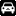.

Now let's save our effect as a DLL file: File-> Save as DLL... Almost all information is in the dialog box, which presented to you. Click the Select icon link and select the icon. Click the Build button - your new effect is ready!

The user interface of our new effect looks like this.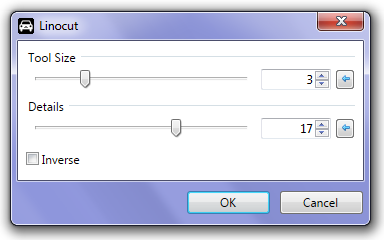And here is the result of the effect work when the checkbox Inverse is marked.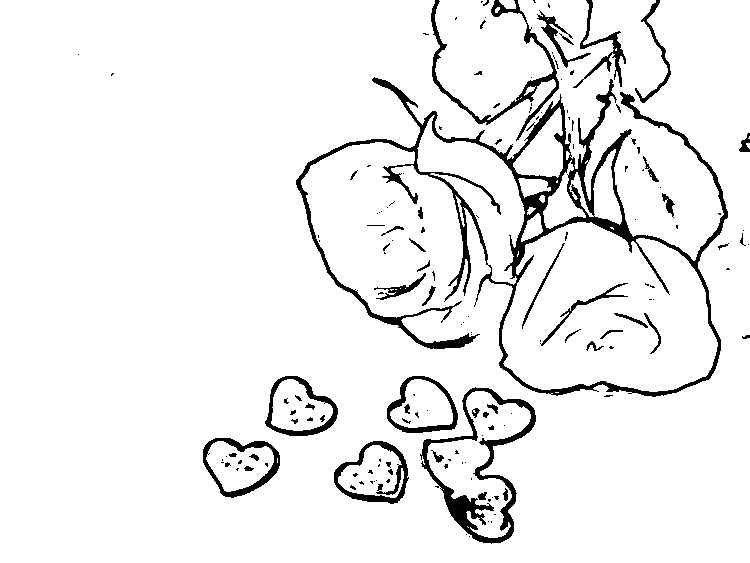I hope that this has not caused you difficulties.

Вариант этой темы на русском языке смотрите здесь.

•1
##### Share on other sites

I'm loving this series of tutorials ReMake!  Thank you.

•1ebook: Mastering Paint.NET | resources: Plugin Index | Stereogram Tut | proud supporter of Codelab
plugins: EER's Plugin Pack | Planetoid | StickMan | WhichSymbol+ | Dr Scott's Markup Renderer | CSV Filetype | dwarf horde plugins: Plugin Browser | ShapeMaker

##### Share on other sites

I'm loving this series of tutorials ReMake!  Thank you.

You are welcome!

I'm glad You like these tutorials. Thank You.

Edited by ReMake

## Join the conversation

You can post now and register later. If you have an account, sign in now to post with your account.×   Pasted as rich text.   Paste as plain text instead

Only 75 emoji are allowed.

×   Your previous content has been restored.   Clear editor

×   You cannot paste images directly. Upload or insert images from URL.

×
×